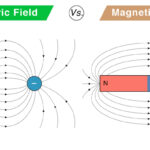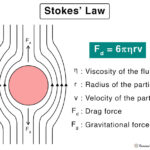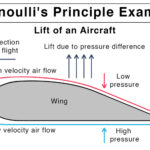Home / Physics / Weak Nuclear Force

# Weak Nuclear Force

## What is Weak Nuclear Force

The weak nuclear force, or weak interaction, is one of the four fundamental forces of nature – the other three being the strong nuclear force, electromagnetic force, and gravitational force. Unlike the other forces that hold matter, the weak nuclear force is responsible for the disintegration of matter. It is observed during the radioactive decay of a few nuclei. It is the least observed fundamental interaction but is the best understood.

History and Discovery of the Weak Nuclear Force

The weak nuclear force was predicted by physicists Steven Weinberg, Abdus Salam, and Sheldon Glashow in 1972 and discovered in 1983 at CERN, Geneva.

## Strength and Range of the Weak Nuclear Force

The weak nuclear force works only at the sub-atomic level, i.e., inside the nucleons, with a concise range, approximately 10-18 m. It is weaker than the strong nuclear force and the electromagnetic force but more potent than gravity.

## What does the Weak Nuclear Force do?

It is responsible for powering stars and creating new elements. Much of the radiations present in the universe can be explained through the weak interaction. The weak force occurs when there is beta decay, especially during a neutron’s transformation into a proton and vice versa. During this process, a down quark disappears, and an up quark is produced. The weak force is responsible for the fusion of neutrons and protons to form a deuteron. It is significant in nucleosynthesis and stellar interiors.

The theory describing its behavior of weak interaction and effects is often called quantum flavourdynamics (QFD). Particles interact through this weak interaction by exchanging force carriers, known as W+, W, and Z bosons. They are known as intermediate vector bosons.

## Properties of Weak Nuclear Force

Here are some facts and characteristics of the weak nuclear force.

• Exchange force – neither attractive nor repulsive
• Short-range (~10-18 m)
• Non-central and non-conservative
• Does not follow product law and the inverse-square law
• A million times weaker than the strong nuclear force

## Examples of Weak Nuclear Force

1. Beta Decay

An example of the weak nuclear force is converting a neutron into a proton and vice versa, resulting in beta (minus) decay. During this process, a neutron is replaced by a proton, an electron, and an antineutrino. This equation is represented by,

0n11p1 + -1β0 + νe (antineutrino)

Similarly, a proton decays into a neutron, a positron, and a neutrino in the following way,

1p10n1 + +1β0 + νe (neutrino)

2. Electron Capture

Electron capture, or K-capture, is the process through which protons transform into neutrons. This reaction occurs when there is an excess number of protons relative to neutrons. An electron from the inner shell falls into the nucleus. The products are the daughter nucleus, with one less atomic number and a neutrino.

An example of electron capture is the decay of krypton-81 into bromine-81.

81Kr36 + e81Br35 + νe

3. Nuclear Fusion

In a nuclear fusion, two protons are smashed together with enough energy to overcome the electrostatic repulsion, which results in an unstable 2He. One of the protons undergoes beta decay mediated by the weak force.  A series of reactions follows, involving the formation and fusion of the intermediate 3He into a stable 4He nucleus. The overall reaction is,

4 1p1+ 2 e4He + 2 νe

Article was last reviewed on Monday, April 12, 2021

### Related articlesElectric Field vs. Magnetic FieldHooke’s LawStokes’ LawBernoulli’s Principle and Equation